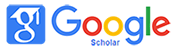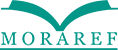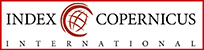### PENERAPAN MODEL PROBLEM BASED LEARNING TERHADAP KEMAMPUAN PEMECAHAN MASALAH MATEMATIK SISWA SEKOLAH DASAR

Nia Apriani, Rayi Siti Fitriani

#### Abstract

This research starts from the problem of the students' low mathematical problem solving abilities so that it influences learning outcomes under the Minimum Completion Criteria (KKM), namely 6. An alternative solution to improve learning outcomes is by applying a problem based learning model. The method used in this study is a quantitative method with a quasi-experimental type. The design of this study uses Nonequivalent Control group Design. The population taken was all fifth grade students of SDN 2 Nagrikidul. The sample taken is class 5A as an experiment and Class 5B as a control. The sampling technique used is saturated sampling, where all of these techniques are used as samples in the study. The data collection technique uses objective tests, while the instruments used are tests in the form of essays. The results showed that there was an influence on the ability of mathematical problem solving between the experimental groups by applying the problem based learning model and the control group with the direct intruction model. This is evidenced from the results of the t-test at the 5% significance level obtained by the calculated significance of 0.939 <0.05. Whereas for the increase, the results of the t-test at the 5% significance level obtained a calculated significance lower than 0.05, namely 0.02 <0.05, so that it can be stated that learning with the Problem Based Learning model has an effect on improving mathematical problem solving skills.

#### Keywords

problem based learning; mathematic problem solving

#### Full Text:

PDF

DOI: http://dx.doi.org/10.32934/jmie.v3i1.100

### Refbacks

• There are currently no refbacks.